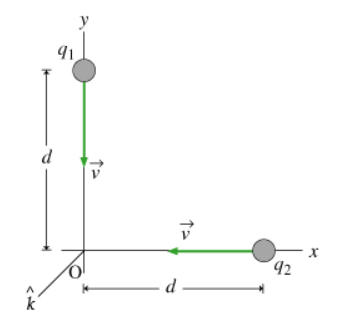# Problem: Two point charges, with charges q1 and q2, are each moving with speed v toward the origin. At the instant shown q1 is at position (0, d) and q2 is at (d, 0). (Note that the signs of the charges are not given because the magnitudes of the forces between the charges do not depend on it.) Part A. What is the magnitude of the electric force between the two charges? Express F in terms of q, d and ε0Part B. What is the magnitude of the magnetic force on q2 due to the magnetic field caused by q1? Express the magnitude of the magnetic force in terms of q, v, d and μ0Part. C Assuming that the charges are moving nonrelativistically (u &lt; c), what can you say about the relationship between the magnitudes of the magnetic and electrostatic forces? a. The magnitude of the magnetic force is greater than the magnitude of the electric force.b. The magnitude of the electric force is greater than the magnitude of the magnetic force.c. Both forces have the same magnitude.

###### FREE Expert Solution

The magnitude of electric force:

$\overline{){\mathbf{F}}{\mathbf{=}}\frac{\mathbf{1}}{\mathbf{4}\mathbf{\pi }{\mathbf{\epsilon }}_{\mathbf{0}}}\frac{{\mathbf{q}}_{\mathbf{1}}{\mathbf{q}}_{\mathbf{2}}}{{\mathbf{r}}^{\mathbf{2}}}}$

Magnetic field due to a moving charge:

$\overline{){\mathbf{B}}{\mathbf{=}}\frac{{\mathbf{\mu }}_{\mathbf{0}}\mathbf{q}\mathbf{v}\mathbf{×}\mathbf{r}}{\mathbf{4}\mathbf{\pi }{\mathbf{r}}^{\mathbf{3}}}}$

Part A

The distance between the two charges is given by sqrt(2)d

93% (308 ratings)###### Problem Details

Two point charges, with charges q1 and q2, are each moving with speed v toward the origin. At the instant shown q1 is at position (0, d) and q2 is at (d, 0). (Note that the signs of the charges are not given because the magnitudes of the forces between the charges do not depend on it.)

Part A. What is the magnitude of the electric force between the two charges? Express F in terms of q, d and ε0

Part B. What is the magnitude of the magnetic force on qdue to the magnetic field caused by q1? Express the magnitude of the magnetic force in terms of q, v, d and μ0

Part. C Assuming that the charges are moving nonrelativistically (u < c), what can you say about the relationship between the magnitudes of the magnetic and electrostatic forces?

a. The magnitude of the magnetic force is greater than the magnitude of the electric force.
b. The magnitude of the electric force is greater than the magnitude of the magnetic force.
c. Both forces have the same magnitude.